Courses

# Ampere's Law and its Applications Class 12 Notes | EduRev

## Class 12 : Ampere's Law and its Applications Class 12 Notes | EduRev

The document Ampere's Law and its Applications Class 12 Notes | EduRev is a part of the Class 12 Course Physics For JEE.
All you need of Class 12 at this link: Class 12

7. Ampere's law

This law is useful in finding the magnetic field due to currents under certain conditions of symmetry. Consider a closed plane curve enclosing some current-carrying conductors.

The line integral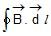taken along this closed curve is equal to m0 times the total current crossing the area bounded by the curve.

i.e.,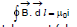...(8)

where i = total current (algebraic sum) crossing the area.

As a simple application of this law, we can derive the magnetic induction due to a long straight wire carrying current i.

Suppose the magnetic induction at point P, distance R from the wire is required.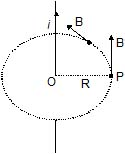Draw the circle through P with centre O and radius R as shown in figure.

The magnetic induction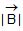at all points along this circle will be the same and will be tangential to the circle, which is also the direction of the length element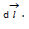Thus,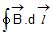=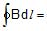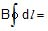B × 2 πR

The current crossing the circular area is i.

Thus, by Ampere's law, B × 2πR = μ0i

⇒ B =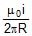Note : -

Line integral is independent of the shape of path and position of wire with in it.

The statement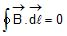does not necessarily mean that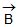= 0 everywhere along the path but only that no net current is passing through the path.

Sign of current : The current due to which is produced in the same sense as (i.e. ) positive will be taken positive and the current which producesin the sense opposite to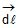will be negative.

Ex.11 Find the value of for the loops L1, L2, L3 in the figure shown. The sense of is mentioned in the figure.

Sol. for L1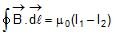here I1 is taken positive because magnetic lines of force produced by I1 is anti clockwise as seen from top. I2 produces lines ofin clockwise sense as seen from top. The sense of is anticlockwise as seen from top.

for L2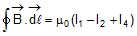for L3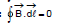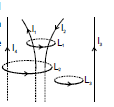7.1 Uses of Ampere's current law : To find out magnetic field due to infinite current carrying wire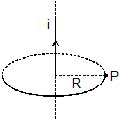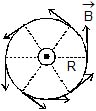By B.S.L.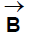will have circular lines.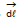is also taken tangent to the circle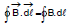Therefore, θ = 0° so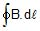= B 2πR (Therefore, B = const.)

Now by amperes law :

B 2πR = μ0I

Therefore, B =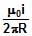7.2 Hollow current carrying infinitely long cylinder : (I is uniformly distributed on the) whole circumference

(i)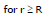By symmetry the amperian loop is a circle.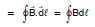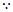θ = 0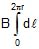B = const.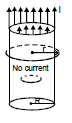⇒ B =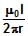(ii) r < R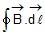=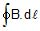= μ0 (0)

= B(2πr) = 0

⇒ Bin = 0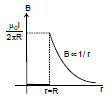Graph

7.3 Solid infinite current carrying cylinder :

Assume current is uniformly distributed on the whole cross section area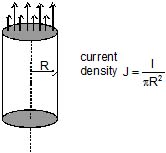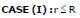taken an amperian loop inside the cylinder. By symmetry it should be a circle whose centre is on the axis of cylinder and its axis also coincides with the cylinder axis on the loop.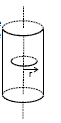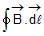==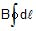= B . 2πr =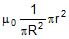B =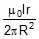=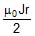⇒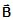=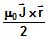Case (II) :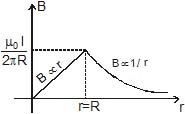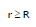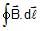== B.(2pr) = m0. I

⇒ B =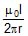also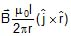=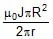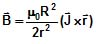Ex.12 Consider a coaxial cable which consists of an inner wire of radius a surrounded by an outer shell of inner and outer radii b and c respectively. The inner wire carries an electric current i0 and the outer shell carries an equal current in opposite direction. Find the magnetic field at a distance x from the axis where (a) x < a, (b) a < x < b (c) b < x < c and

(d) x > c. Assume that the current density is uniform in the inner wire and also uniform in the outer shell.

Sol.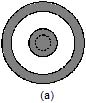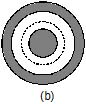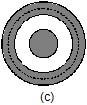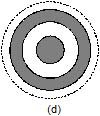A cross-section of the cable is shown in figure. Draw a circle of radius x with the centre at the axis of the cable. The parts a, b, c and d of the figure correspond to the four parts of the problem. By symmetry, the magnetic field at each point of a circle will have the same magnitude and will be tangential to it. The circulation of B along this circle is, therefore,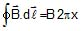in each of the four parts of the figure.

(a) The current enclosed within the circle in part b is i0 so that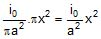Ampere's law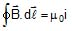gives

B. 2πx =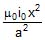or, B =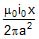The direction will be along the tangent to the circle.

(b) The current enclosed within the circle in part b is i0 so that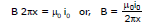(c) The area of cross-section of the outer shell is πc2 - πb2. The area of cross-section of the outer shell with in the circle in part c of the figure is πx2 - πb2.

Thus, the current through this part is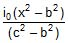. This is in the opposite direction to the current i0 in the inner wire. Thus, the net current enclosed by the circle is

inet = i0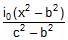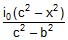From Ampere's law,

B 2px =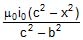or, B =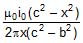(d) The net current enclosed by the circle in part d of the figure is zero and hence

B 2px = 0 or, B = 0.

Ex.13(a)Figure shows a cross-section of a large metal sheet carrying an electric current along its surface. The current in a strip of width dl is Kdl where K is a constant. Find the magnetic field at a point P at a distance x from the metal sheet.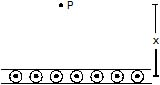Sol. Consider two strips A and C of the sheet situated symmetrically on the two sides of P (figure). The magnetic field at P due to the strip A is B0 perpendicular to AP and that due to the strip C is Bc perpendicular to CP. The resultant of these two is parallel to the width AC of the sheet. The field due to the whole sheet will also be in this direction. Suppose this field has magnitude B.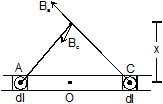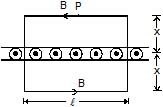The field on the opposite side of the sheet at the same distance will also be B but in opposite direction. Applying Ampere's law to the rectangle shown in figure.

2B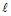= μ0 Kor, B =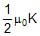Note that it is independent of x.

Ex.13 (b) A cylinder of radius R1 have cylindrical cavity of radius R2 as shown in the figure and have current density J (down ward). Find Magnetic field when

(i) r > R1 (on x-axis towards right)

(ii) r < R1 (on x-axis towards left)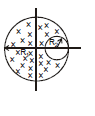(iii) when point is in cavity

Sol. (i) r > R1 (on x-axis towards right)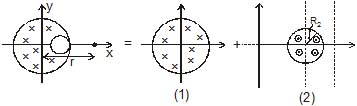Magnetic field due to big cylinder = B1

Magnetic field due to smaller cylinder = B2

Br = B1 + B2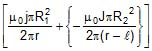(ii) r < R1 (on x-axis) towards left of centre Magnetic field due to big cylinder

B1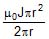Magnetic field due to smaller cylinder

B2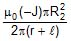So net Magnetic field

Offer running on EduRev: Apply code STAYHOME200 to get INR 200 off on our premium plan EduRev Infinity!

## Physics For JEE

187 videos|516 docs|263 tests

,

,

,

,

,

,

,

,

,

,

,

,

,

,

,

,

,

,

,

,

,

;